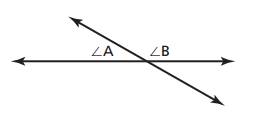# User Forum

Subject :IMO    Class : Class 7

If the value of angle A = x + 2 and the value of angle be = 2x + 4, then the measurement of angle A will beA30 degree
B60 degree
C105 degree
DCannot be calculated with the given data

I THINK THE ANSWER SHOULD BE 60 DEGREES THAT IS OPTION B

## Ans 1:

Class : Class 9
ANGLE A +ANGLE B=180 DEGREE X+2+2X+4=180 DEGREE 3X+6=180 3X=180-6 3X=174 X=174/3 X=58 ANGLE A=X+2 =58+2 =60 DEGREE

## Ans 2:

Class : Class 7
A and B are supplementary angles(linear pair). So a+b=180. If we solve this equation, x+2+2x+4=180, we get A is 60.

## Ans 3:

Class : Class 8
The correct answer indeed is B

Class : Class 7
60 degree

## Ans 5:

Class : Class 7
Ans is B 60Degree

Class : Class 9
You see that